# Bicategory(2)

(diff) ← Older revision | Latest revision (diff) | Newer revision → (diff)

A category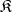in which subcategories of epimorphisms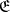and of monomorphisms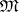have been distinguished such that the following conditions are met:

1) all morphismsinare decomposable into a product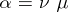, where,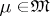;

2) if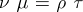, where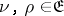,, then there exists an isomorphismsuch that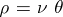, and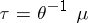;

3)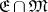coincides with the class of isomorphisms in the category.

The epimorphisms in(the monomorphisms in) are called the permissible epimorphisms (monomorphisms) of the bicategory.

The concept of a bicategory axiomatizes the possibility of a decomposition of an arbitrary mapping into a product of a surjective and an injective mapping. The category of sets, the category of sets with a marked point and the category of groups are bicategories with a unique bicategorical structure. In the category of all topological spaces and in the category of all associative rings there are proper classes of different bicategorical structures.

How to Cite This Entry:
Bicategory(2). Encyclopedia of Mathematics. URL: http://encyclopediaofmath.org/index.php?title=Bicategory(2)&oldid=11447
This article was adapted from an original article by M.Sh. Tsalenko (originator), which appeared in Encyclopedia of Mathematics - ISBN 1402006098. See original article